Math Quiz For Everybody
Question 1 of 10
What is the remainder when we divide 30 by 4?
Question 2 of 10
What is the distance around a shape called?
Question 3 of 10
What is 3/4 in decimal form?
Question 4 of 10
What is the perimeter of a circle also called?
Question 5 of 10
What does the 8 mean in the number 582?
Question 6 of 10
What are the factors in this problem: 2 x 8 = 16?
Question 7 of 10
What is 3291 rounded to the nearest 1000?
Question 8 of 10
A mixed number contains what two elements?
Question 9 of 10
In the fraction 3/4, which number is the denominator?
Question 10 of 10
What does the symbol ">" mean in math?Try another one of our quizzes
Science and Nature Quiz
How many question can you answer correctly?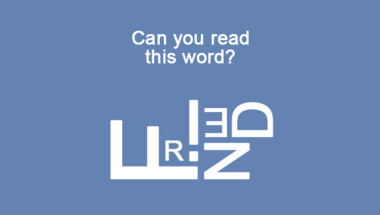Trivia Quiz with 10 mixed ques
The last 3 questions will be very hard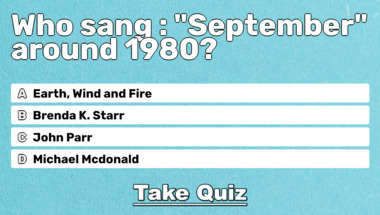Hard Song Quiz About 1977
You Wont Even Get 4 Correct AnswersGeneral Knowledge For Trivia E
Trust us, the last 2 questions will haunt you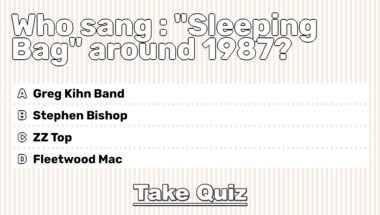10 mixed categories questions
How's your trivia brain doing?History Quiz
10 trivia questions for everyoneGeneral Trivia Quiz
How smart are you really?Very Hard General Trivia Quiz
10 Impossible QuestionsGeneral Trivia Quiz
10 mixed questions for you to have fun with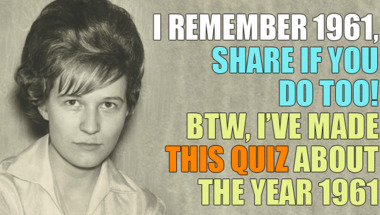General Trivia Quiz
10 questions to test your knowledgeQuiz about the years 1960-1969
10 questions to test your knowledge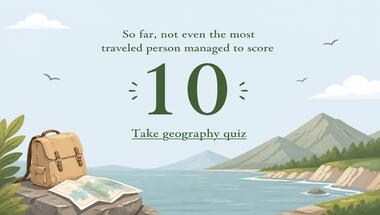Quiz : Songs of the 1978 hit l
How many correct will you get?1976 Hit List Quiz
10 songs. 10 questions. Can you beat it?Mixed Knowledge Quiz
10 questions to test your knowledge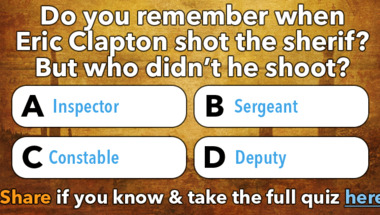70s Music Quiz
10 hard songs to guess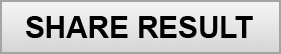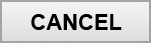Copyright 2019 - Wicked Media ApS